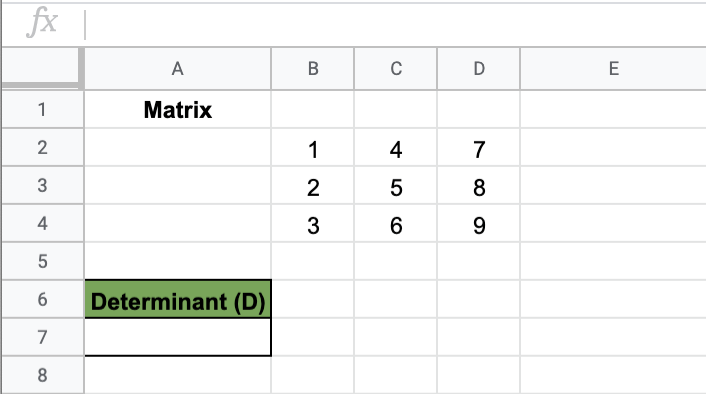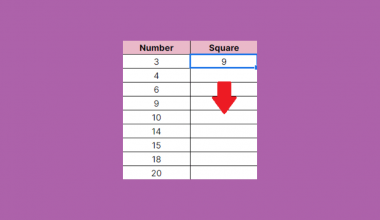# How to Use MDETERM Function in Google Sheets

The MDETERM function in Google Sheets is used to find the matrix determinant of a square matrix.

The `MDETERM` function is used to find the matrix determinant. A matrix determinant is a special number in mathematics and it is an input that helps you determine various qualities of a matrix.

It is very easy to manually calculate the matrix determinant of a 2×2 matrix. But as soon as you encounter a 3×3 matrix, it’s increasingly difficult to remember the formula and correct order of calculations. Thankfully, there is a readily available way to calculate this with the helpful MDETERM function in Google Sheets.

Finding the matrix determinant is useful in linear algebra and calculus. It is used to solve systems of equations, determine the change in area and volume of a matrix, and changing variables for integrals. For example, it is useful for everyday problems to case studies in science and finance.

Let’s look an at example.

Matrix determinants are usually used to solve systems of equations in linear algebra with multiple variables.

You need to compare cell phone plans according to your budget. Included in your consideration are the following: the monthly base fee, and additional fees according to gigabytes of data usage and according to minutes of calls made. You might want to compare the amount of gigabyte or call usage that might make the plans have the same monthly costs and what should be chosen as the most efficient plan for you.

The `MDETERM` function needs you to set up a square matrix, set by the coefficients of your system of equations.

You will need a complete set of matrix determinants to figure out the x and y.

## The Anatomy of the MDETERM Function in Google Sheets

The syntax of the `MDETERM` function is as follows::

`=MDETERM(square_matrix)`

Let’s have a look at each part of the function to understand what is going on here:

• `=` is the equals sign that starts off any function in Google Sheets.
• `MDETERM` is the name of our function. We need a range of numbers as its input.
• `square_matrix` is the input of the function. Choose an array of numbers that covers your entire matrix, of which you want to find the square determinant.

Make sure that you input a complete matrix within the `MDETERM` Function. This means the whole matrix, with an equal number of rows and columns.

## A Real Example of Using the MDETERM Function

Let’s look at the example below to see how to use `MDETERM` function in Google Sheets.

In algebra, systems of equations can be solved in various ways. One of the popular solutions involves using matrix determinants to find the values of x, y, and other variables in the system.

Google Sheets is equipped with a convenient and easy to use way to find these determinants. The larger the square matrix, the more complex the calculations are – if you are solving 3×3, 4×4, 5×5, and so on sizes of square matrices, this is the perfect solution for you.

#### Finding the Square Determinant of a Matrix in Google Sheets

This is a simple linear equation problem. We want to find x and y using the square determinant of the matrix.The function takes only one argument. So in the equation, it will look like:

`=MDETERM(C6:D7)`

As a result, we get -2.

To completely solve the system of equations, we also get the determinant of the x and y matrix.

To get the determinant of x, we use a matrix that replaces the coefficients of x with the numbers on the right side of the equation. Given this, we use the equation:

`=MDETERM(C10:D11)`

As a result, we get -13.

To get the determinant of y, we use a matrix that replaces the coefficients of y with the numbers on the right side of the equation. Given this, we use the equation:

`=MDETERM(C14:D15)`

As a result, we get 3.

You can then use the given formulas for x and y to find out that x = 6.5 and y = – 1.5 to solve the system of equations.

This simple problem can be practiced to perfection. Use the link below:

## How to Use the MDETERM Function in Google Sheets

In this section, we will show you a step-by-step process on how to use the `MDETERM` function in Google Sheets.

Finding the Square Determinant in Google Sheets

1. To begin, click on a cell to make active, which you would like to display the determinant of the matrix. For the guide. The determinant will be in Cell A6, where I will display the determinant.1. Next, type the equal sign ‘=’ to start writing the function. Follow this with “MDETERM” or “mdeterm” – Google Sheets functions are not case sensitive so either is fine.
2. The auto-suggest box will create a drop-down menu. Select the `MDETERM` function by clicking it. It is the first to pop up on the list, but take care to choose the correct function.1. After the opening bracket ‘(‘, you will add the range attribute. You can input the cell addresses one by one, but it is safer to list down the range or drag your cursor over the entire matrix instead. You will notice that there will be a preview of the result when you fill in the range.1. After filling in the range, press Enter to complete the function. A closing bracket will automatically be added to the function, and it will immediately display the resulting matrix determinant.1. You can now see the matrix determinant of the initial array.Given a practical problem where you should completely solve the system of equations, use `MDETERM` to find the matrix determinants of the other variables.

And there you have it – you can now use the MDETERM function in Google Sheets together with the other numerous Google Sheets formulas to create even more effective formulas.Our goal this year is to create lots of rich, bite-sized tutorials for Google Sheets users like you. If you liked this one, you'll love what we are working on! Readers receive ✨ early access ✨ to new content. There will be no spam and you can unsubscribe at any time.

##### You May Also Like## How to Convert Month Name to Number in Google Sheets

Knowing how to convert month name to number in Google Sheets is useful if you have your month…## How to Use ISEMAIL Function in Google Sheets

The ISEMAIL function in Google Sheets is used to determine if a value is a valid e-mail address.…## How to Create Candlestick Chart in Google Sheets

A candlestick chart is a visualization tool available in Google Sheets that helps track movements in the value…## How to Copy a Formula Down an Entire Column in Google Sheets

You can easily copy a formula down an entire column in Google Sheets to automate your work and…